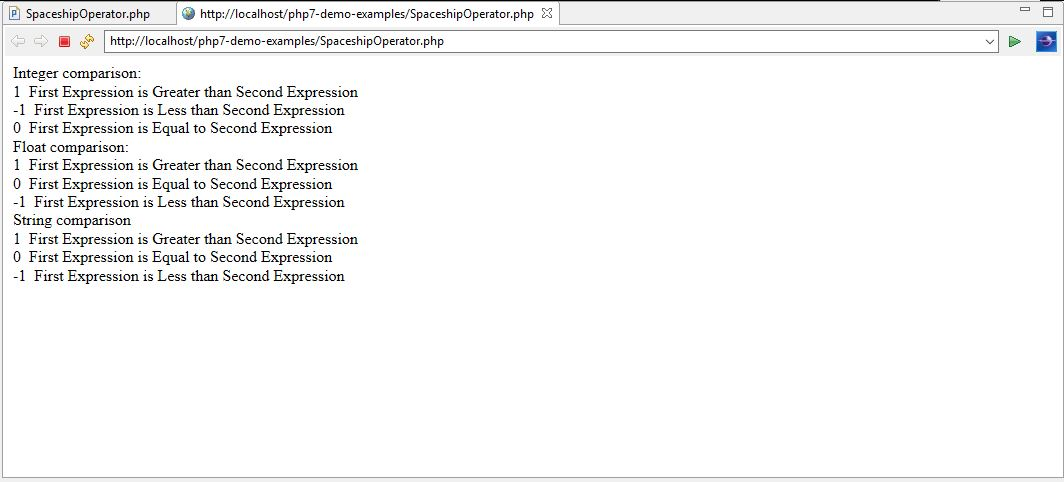# Learn About Null Coalescing and Spaceship Operators in PHP7

0
4592Null Coalescing and Spaceship Operators
In the last chapter, we discussed one of the new PHP 7 features, scalar and return type declarations with examples. In this chapter, we are going to learn about null coalescing and spaceship operators, which are two new operators added to PHP 7.

Null Coalescing Operators (??)
In PHP 7, Null Coalescing Operator is denoted by a double question mark (??). Previously, PHP parser had a ternary operation which used to work in conjunction with the isset () function. In PHP 7, Null coalescing operator returns the first operand if its value exists and is not NULL otherwise it will return the value present in the second operand.  Below is the syntax of this operator.
(Operand 1) ?? (Operand 2)

Chaining Null Coalescing operator (??…??)
Null Coalescing operator can be used in a chain format,one after the other following the same logic as above (returns the first operand if its value exists and is not null otherwise returns the second operand). If first operand has a not null value, then it will be returned otherwise null coalescing operator will move to the second operand and checks its value, return it if the value exists and is not null otherwise the operator will move to the third operand and so on. This methodology in PHP 7 is known as chaining NULL coalescing operator. Below is the syntax of this chaining operator.
(Operand 1) ?? (Operand 2) ?? (Operand 3)

In the following examples, we are going to demonstrate the use of Null coalescing operator introduced in PHP 7. Also, we will compare this operator against Chaining Null Coalescing operator and ternary operation.

PHP 7 Example for Null Coalescing Operators (??)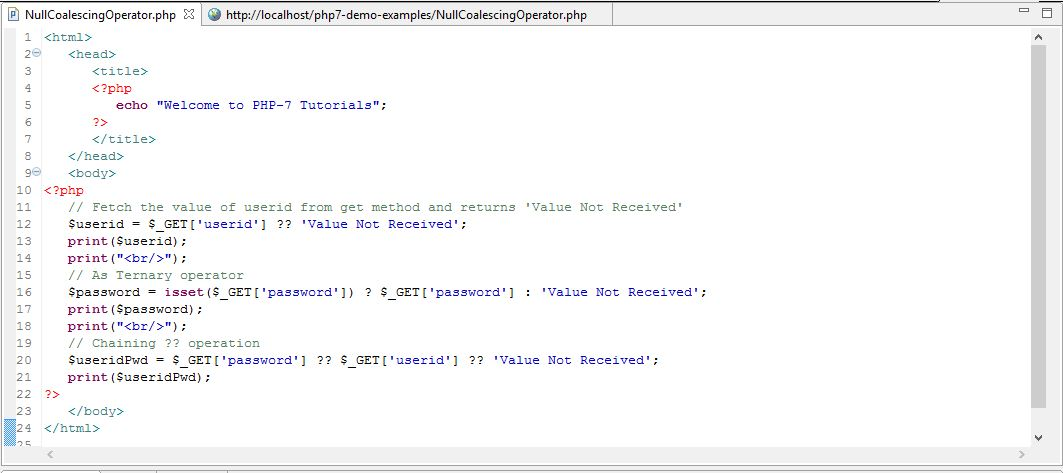Explanation of PHP 7 code

• First, we have used the NULL coalescing operator on the PHP GET method fetching the value for the ‘userid’ form variable from the URL. Therefore, if GET method is able to fetch the value of the ‘userid’ from the URL and it is not NULL then the value of \$_GET [‘userid’] will be returned to the variable \$userid using this operator, otherwise second operand which is ‘Value Not Received’ will be returned.
• Second, we have used a ternary operator in conjunction with isset () function. If the condition in isset (\$_GET [‘password’]) function is true or value is set for ‘password’ then it will return the first operand that is \$_GET [‘password’] into variable ‘\$password’, otherwise it will return the second operand which is ‘Value Not Received’.
• Last, we have used chained NULL coalescing operator where first operand is \$_GET [‘password’], second operand is \$_GET [‘userid’] and third operand is ‘Value Not Received’. This operator in chained mode will behave in the following way.
• If first operand exists and not NULL, then that operand will be returned.
• If first operand does not exist and is NULL. It will pass on to the second operand. If it exists and not NULL, then it will return this operand.
• If in the above case, the second operand does not exist and is NULL, then it will return the third operand to the variable \$password.

Output for Case 1:

URL is http://localhost/php7-demo-examples/NullCoalescingOperator.php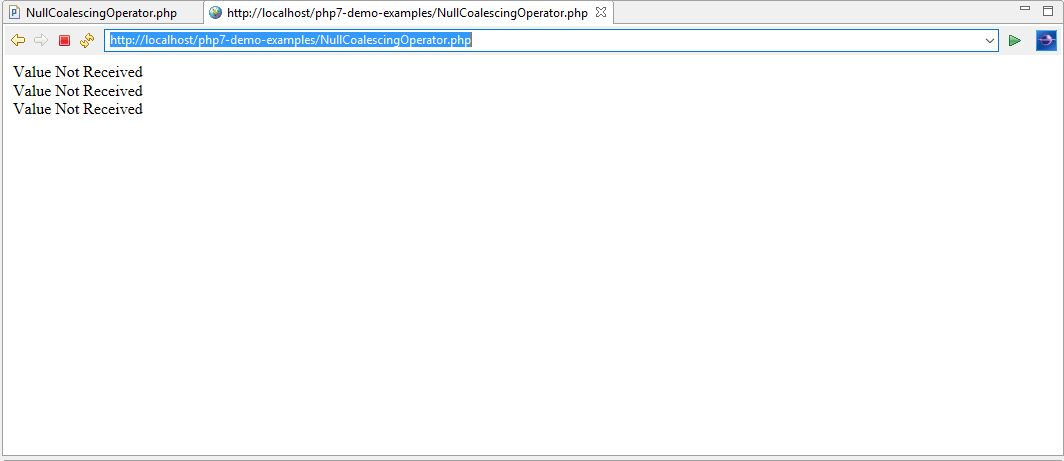This is the expected output as the URL does not contain the values for ‘userid’ and ‘password’ variables.

Output for Case 2:
URL is http://localhost/php7-demo-examples/NullCoalescingOperator.php?userid=PHP7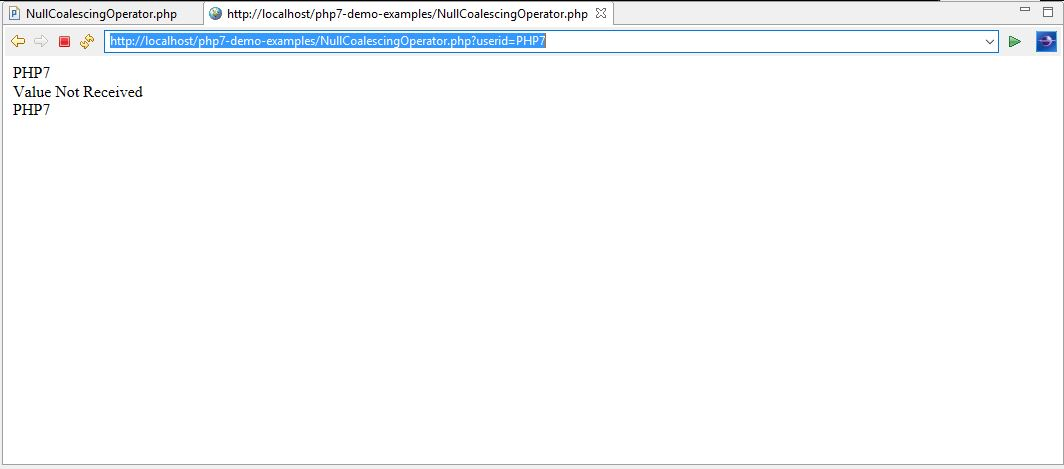As explained before, this is the expected output as we have passed the value for userid = PHP7 but not passed the value for ‘password’ form variable.

Output for Case 3: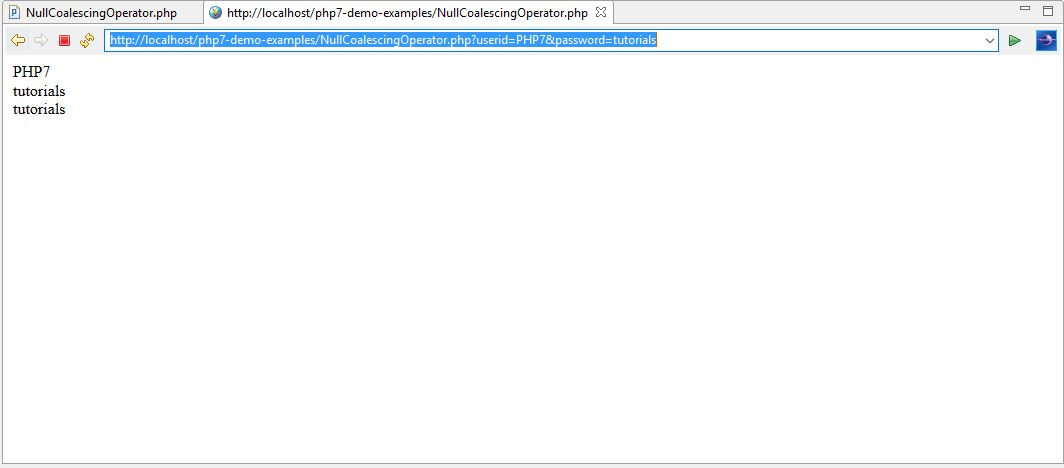This is the expected output if we pass the values for both ‘userid’ and ‘password’ form variables.

Spaceship Operators
In PHP 7, the Spaceship Operator is denoted by (<=>) symbol. It is also a new feature in PHP 7. This operator is used for comparing two expressions or operands and return result as shown below.

 S No. First expression <=> Second expression Return Value 1 Less than -1 2 Equal to 0 3 Greater than 1

We are going to understand the use of this operator using following example.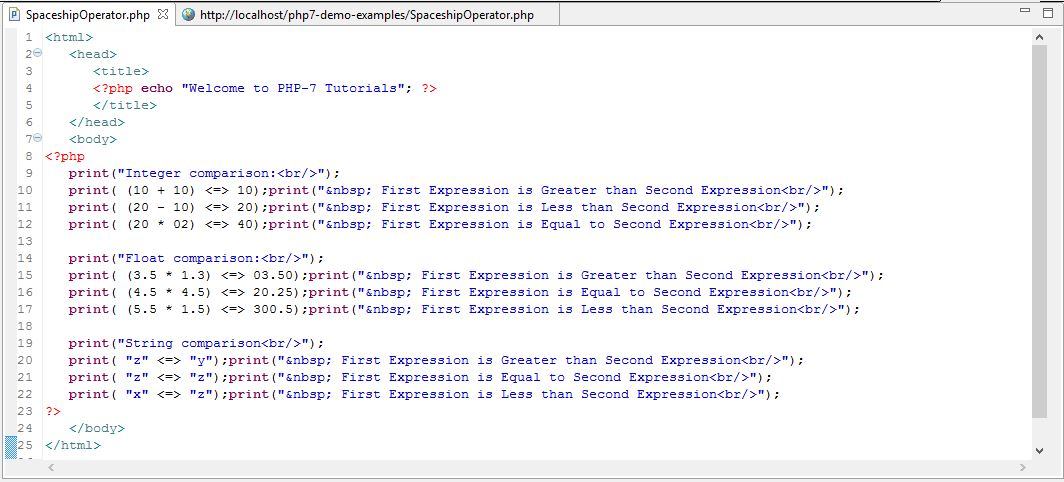Explanation of the Code

• Integer Comparison: Here, we are comparing the integers present in the first expression and second expression with the spaceship operator in between. Following three scenarios are possible.
• If the integer present in the first expression is greater than the integer present in the second expression, the spaceship operator will return 1.
• If the integer present in the first expression is equal to the integer present in the second expression, the spaceship operator will return 0.
• If the integer present in the first expression is less than the integer present in the second expression, the spaceship operator will return -1.
• Float Comparison: Here, we are comparing floating number (decimals) present in the first expression and second expression with the spaceship operator in between. Following three scenarios are possible.
• If the floating number present in the first expression is greater than the floating number present in the second expression, the spaceship operator will return 1.
• If the floating number present in the first expression is equal to the floating number present in the second expression, the spaceship operator will return 0.
• If the floating number present in the first expression is less than the floating number present in the second expression, the spaceship operator will return -1.
• String Comparison: Here we are comparing the strings present in the first expression and second expression with the spaceship operator in between. Following three scenarios are possible.
• If the string present in the first expression is greater than the string present in the second expression, the spaceship operator will return 1.
• If the string present in the first expression is equal to the string present in the second expression, the spaceship operator will return 0.
• If the string present in the first expression is less than the string present in the second expression, the spaceship operator will return -1.

Output of the Spaceship PHP 7 Program

Given below is the output of the PHP 7 program that demonstrates comparison of two integer, floating point number and string expressions.